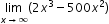# MA.912.C.1.5

Find limits at infinity.

### Examples

Example: Find.
General Information
Subject Area: Mathematics (B.E.S.T.)
Strand: Calculus
Status: State Board Approved

## Related Courses

This benchmark is part of these courses.
1202300: Calculus Honors (Specifically in versions: 2014 - 2015, 2015 - 2022, 2022 and beyond (current))

## Related Access Points

Alternate version of this benchmark for students with significant cognitive disabilities.

## Related Resources

Vetted resources educators can use to teach the concepts and skills in this benchmark.

## Text Resource

No Limits For Usain:

This informational text resource is intended to support reading in the content area. The text uses the story of Usain Bolt and his quest to reduce his world record in the 100 meter race to raise the question of whether there is a limit to his—or any human's—athleticism. The article uses number series, limits, and convergent and divergent series to prove that, hypothetically at least, a world record will go on reducing beyond any limit. A logistic curve is shown to model the data.

Type: Text Resource

## Tutorials

Limits at Positive and Negative Infinity:

In this video we will find the limit of a function as it approaches positive infinity and negative infinity.

Type: Tutorial

More Limits at Infinity:

Here we will explore three more functions, and find their limits as they approach infinity.

Type: Tutorial

Limits at Infinity Using Algebra:

Here we will use algebra to find the limit of a function with radicals as it approaches infinity.

Type: Tutorial

Limits with Two Horizontal Asymptotes:

Here we will find the limit of a function as it approaches positive and negative infinity and results in two horizontal asymptotes.

Type: Tutorial

## Student Resources

Vetted resources students can use to learn the concepts and skills in this benchmark.

## Tutorials

Limits at Positive and Negative Infinity:

In this video we will find the limit of a function as it approaches positive infinity and negative infinity.

Type: Tutorial

More Limits at Infinity:

Here we will explore three more functions, and find their limits as they approach infinity.

Type: Tutorial

Limits at Infinity Using Algebra:

Here we will use algebra to find the limit of a function with radicals as it approaches infinity.

Type: Tutorial

Limits with Two Horizontal Asymptotes:

Here we will find the limit of a function as it approaches positive and negative infinity and results in two horizontal asymptotes.

Type: Tutorial

## Parent Resources

Vetted resources caregivers can use to help students learn the concepts and skills in this benchmark.